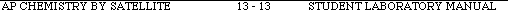### ContentsReturn toIndex of ExperimentsPre-lab QuestionsExperimentPost-lab Questions

EXPERIMENT 13: PHOTOMETRIC DETERMINATION OF AN EQUILIBRIUM CONSTANT

The following preparatory questions should be answered before coming to class. They are intended to introduce you to several ideas important to aspects of the experiment. You must turn-in your work to your instructor before you will be allowed to begin the experiment. Be sure to bring a calculator and paper to laboratory.l. The equilibrium constant, Kc, for the reaction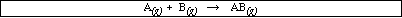is 115 at 25 ºC. If 1.580 x 10-1 mol of A and 4.721 x 10-5 mol of B are introduced into a 1.00 liter vessel at 25 ºC, calculate the equilibrium concentration of all species.2. Answer the following questions regarding problem #1:

(a) How does the amount of B reacting compare to the initial amount of B?(b) How does the amount of AB at equilibrium compare to the initial amount of B?(c) What is it about the initial conditions that might explain your answer in 2 (b)?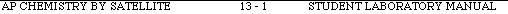3. The equilibrium constant, Kc, for the reactionis unknown at 25 ºC. If 1.934 x 10-1 mol of C and 7.315 x 10-5 mol of D are introduced into a 1.00 liter vessel at 25 ºC, calculate the equilibrium concentration of CD.Discuss any assumptions you made in arriving at your answer.4. (a) This experiment is separated into parts I and II. What are the [Fe3+] and [SCN-] before mixing in each part?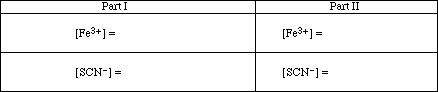(b) Briefly, explain why the concentrations of the Fe3+ and SCN- are different in the two parts of the experiment.(c) How are you going to keep from mixing the wrong combinations when you are doing the experiment?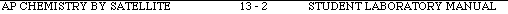EQUIPMENT: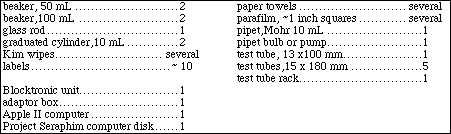Part I. Calibration Line (Note: The concentrations of reagents vary in Part I and Part II)

The purpose of this experiment is to determine the equilibrium constant for a chemical reaction.To begin the experiment obtain 10 mL samples of 0.200 M Fe(NO3)3 and 9.00 x 10-4 M KSCN. Complete the following table: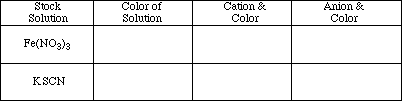Mix the two 10 mL samples and record your observations.

Obs. #1Write the ionic and net ionic equation that best describes the reaction that occurred when the two solutions were mixed. In the net ionic equation indicate the color of each species.

Equ. #1 and #2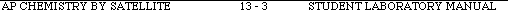The equilibrium constant expression for the net ionic reaction is:

Expl. #1The equilibrium constant, Kc, can be calculated from the equilibrium expression above if the equilibrium concentration of all species are known.In the experiment, known amounts of the reactants Fe3+ and SCN- (thiocyanate ion) will be allowed to react to form the product FeSCN2+. If there were methods of determining the amount of FeSCN2+ at equilibrium, it would be possible to stoichiometrically calculate the equilibrium concentration of the reactants. Substituting into the equilibrium expression above would yield the equilibrium constant.Fortunately, because FeSCN2+ is colored, a light measuring device called a spectrometer can be used to determine the concentration of FeSCN2+ at equilibrium. Consider the color of the FeSCN2+

complex and your experiences in Experiment 11, "Introduction to Blocktronic Spectrometry." Can the Blocktronic spectrometer be used to determine the concentration of this complex in solution? Explain.

Expl. #2:Briefly describe the mathematical relationship introduced in Experiment 11 that will allow us to determine the unknown concentrations.

Expl. #3:Briefly, discuss the importance of the the calibration curve and how it will be used in Part II of this experiment.

Expl. #4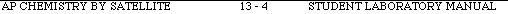Connect the Blocktronic unit to the Apple II computer as directed in Appendix II, Section I. Start running the software as directed in Section II.

A. Preparing Solutions:Place the five 18 x 150 mm test tubes in a test tube rack and clearly label them A through E. Obtain 60 mL of the 0.200 M Fe(NO3)3 solution in a 100 mL beaker, 20 mL of 9.00 x 10-4 M KSCN in a 50 mL beaker and 40 mL of 0.500 MHNO3 in another 50 mL beaker. Write the chemical equations which indicate the ions that are in these three solutions.

Equ. #1, #2, and #3Extreme care must be exercised in preparing solutions A - E to insure that the exact amount of each reagent is added to each test tube. To do this, use Mohr pipets to dispense the specific volume. Follow your instructor's directions on how to properly use a Mohr pipet. (DO NOT MOUTH PIPET.) Practice with the pipet before beginning the mixing of solutions A - E. A few minutes of practice can prevent having to repeat the experiment. If at any time during the preparation of the mixtures a solution in a Mohr pipet turns brownish red, the pipet is contaminated. It must be cleaned and the solution for that test tube prepared again. Suggestion: Add one of the reagents to all the test tubes, clean the Mohr pipet and add another reagent to the test tube, etc.

Pipet into each test tube the amount of each reagent specified in Table I. Carefully mix the solutions so the color of each is homogeneous.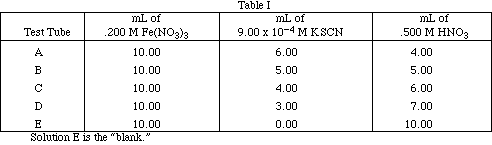B. Calibrating Blocktronic and Measuring Sample Absorption of Solutions A-D.

In the measurements that follow, refer to Appendix II, Sections III and IV as needed for specific instructions on operating the Blocktronic spectrometer:

Calibrate the Blocktronic spectrometer using Solution E as the blank. Be sure to return the blank solution to the 15 x 180 mm test tube after use as you may need to recalibrate the Blocktronic several times before you finish the experiment.

Measure the absorbance of each of the five solutions (Solutions A - E) and record the measured values in Table II. Rinse the test tube thourghly with a small amount of the solution to be tested before filling the tube. Make sure the mark on the test tube is lined up with the mark on the Blocktronic for each reading.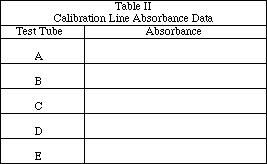Using the data in Table I, complete Table III.
Note: Be sure to take into account the dilution effect.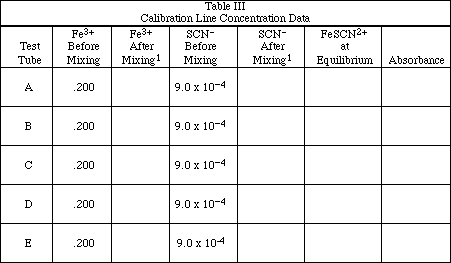Work Space: (Show sample calculation for one of these tubes).Plot the 5 data points on a full sheet of graph paper to obtain a calibration line. The calibration line (Beer's Law Plot) is prepared by plotting absorbance (y-axis) versus [FeSCN2+] (x-axis). Draw the best straight line through the points with a ruler. Calculate the slope and intercept of the line. The calibration line will be needed to complete Table VII.1 After mixing means after combining the three solutions. Calculate the new concentrations in the solutions prior to the reaction occurring.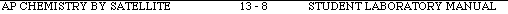EXPERIMENT 13: PHOTOMETRIC DETERMINATION OF AN EQUILIBRIUM CONSTANT

EQUIPMENT: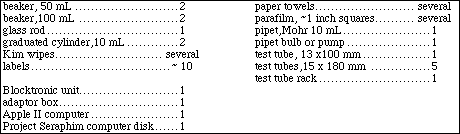Part II. Determination of Kc (Note: The concentrations of reagents vary in Part I and Part II)

A. Preparing Solutions:

Clean the test tubes containing solutions A-D and re-label three of them for solutions F through H. Do not throw away Solution E. Fill each test tube with the amounts of each reagent specified in Table IV and carefully mix each solution so the color is homogeneous. As in Part I, be as accurate as possible when using the Mohr pipet. Be careful to use solutions having the correct concentrations. Do not use the same iron and thiocyanate solutions as in Part I!!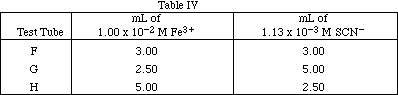Before measuring the absorbances of solutions, recalibrate the Blocktronic with the blank, Solution E. Use the same test tube that was used in Part I. Refer to Appendix II, Sections III and IV for specific instructions for operating the Blocktronic spectrometer.

Measure the absorbances of Solutions F-H and record the data in Table V. Be sure to carefully rinse the sample test tube with the solution to be measured and to wipe off the outside of the test tube before inserting it into the Blocktronic. Make sure the mark on the test tube is lined up with the mark on the Blocktronic. Do not forget to place the box over the top of the unit when making readings.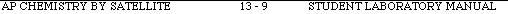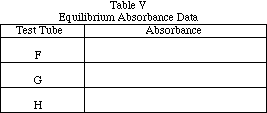Complete Table VI.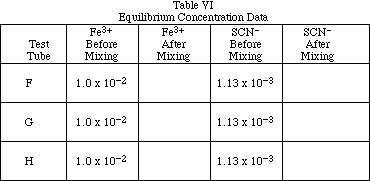Work Space: (Show sample calculation for one of the test tubes).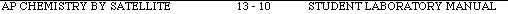Complete the table below. The FeSCN2+ concentration at equilibrium must be obtained from your plot. Explain in words and clearly show (either by drawing on the plot or calculating from the slope and intercept) how these values are obtained.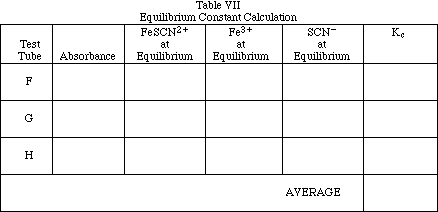Work Space: (Show sample calculation for one of the test tubes). Calculate the average value obtained for Kc and record it in Table VII.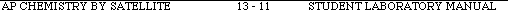Post-lab Questions:Top

Additional problems to be turned in with the laboratory write-up. SHOW ALL CALCULATIONS.

1. In the reaction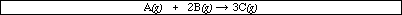initially 1.80 mol of A, 4.20 mol of B and 1.00 mol of C are placed in a 3.00 liter container. Analysis shows 4.50 mol of B at equilibrium. Calculate the equilibrium constant for the reaction.2. In the space below enter the concentrations of Fe3+ and SCN- in test tube 'C'.Fe3+ = _____________ MSCN- = _____________ M

absorbance = _____________When a few drops of Fe3+ are added to test tube 'C' the absorbance of the solution does not change significantly. Briefly, explain this observation.When a few drops of SCN- are added to test tube "C" the absorbance of the solution does change. Briefly, explain this observation.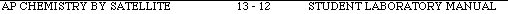3. Consider Problem #1 on page 1 of the experiment. What would the percentage error be if we assumed the equilibrium concentration of AB was equal to the initial concentration of B?The equilibrium constant, Kc, for the reactionis 115 at 25 ºC. If 1.580 x 10-1 mol of A and 4.721 x 10-5 mol of B are introduced into a 1.00 liter vessel at 25 ºC, calculate the equilibrium concentration of all species.### ContentsReturn toIndex of ExperimentsPre-lab QuestionsExperimentPost-lab QuestionsTop